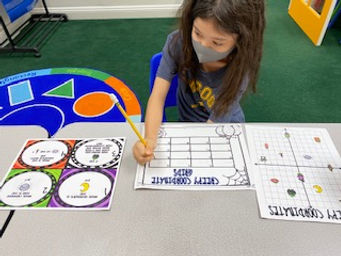## Ms. Dawn

### Target 1​

###### Lesson Type:

Continuation

Number Operation

:

Integer Composition

Recognize a whole number is a multiple of each of its factors.

###### 1:

Determine whether a given whole number in the range of one to 100 is a multiple of a given one-digit number.

3rd

###### Vocabulary:

Multiple, Factor, Pattern, Factor rainbow

Activities:

1. Students used their knowledge of factors to complete a maze of a variety of numbers. Students used "Factor Rainbow" as a strategy.

2. Students used their knowledge of multiples to help identify factors of given numbers.### Home Exploration

###### Guiding Questions:## Absent Students:

### Target 2

:

###### 1:

Understand that the first number in a coordinate pair indicates placement on the x-axis and the second indicates placement on the y-axis.

###### 2:

Become familiar with quadrant II of the coordinate plane and that the first number can be either positive or negative.

3rd

###### Vocabulary:

Coordinates, Coordinate plane, Quadrant, Pair, X-axis, Y-axis, Points, Plot

Activities:

1. We discussed the x-axis and y-axis using all four quadrants. Discussed the positive and negative numbers. Then, we did a few examples and graphed coordinates on the whiteboard.

2. Students viewed and answered task cards with a variety of Halloween coordinate questions.

3. Students played a game called "Candy Hunt." Students needed to plot points, verbally call out plot points, and try and find their opponents candies.### Home Exploration

###### Guiding Questions:### Target 3

:

###### Vocabulary:

Activities:### Home Exploration